# What is 8

What is the perimeter of a rectangle with side lengths of ¾ inch and ⅔?

p =  2.8333

### Step-by-step explanation: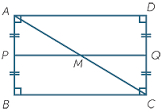Did you find an error or inaccuracy? Feel free to write us. Thank you!Tips to related online calculators
Do you want to convert length units?

#### You need to know the following knowledge to solve this word math problem:

We encourage you to watch this tutorial video on this math problem:

## Related math problems and questions:

• PerimetersA rectangle has a perimeter of 16p centimeters, it had a width of 2p centimeters. Each side of an equilateral triangle is 1/2 the length of the rectangle. Find the total perimeter of the rectangle and the triangle if p=8.
• Photo enlarge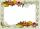Mark wants to enlarge a 4-inch by 6-inch photo so that it has a height of 15inches. Use a ratio table to determine the new width of the photo.
• RectangleThe length of one side of the rectangle is three times the length of the second side. What are the dimensions of the rectangle if its circumference 96 cm?
• Second sideCalculate the length of the other side of the rectangle if its circumference is 60 cm and one side is 10 cm long.
• The perimeter 3The perimeter of a rectangle is 35 cm. The ratio of the length to its width is 3:2. Calculate the dimensions of the rectangle
• Length 7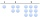Length equals 7/6 inch in width equals 7/9 inches the area is?
• Rectangle JANOThe rectangle has side lengths | JA | = 16cm and | AN | = 12cm. Point S is the center of the JO side and point T is the center of the JA side. Calculate the perimeter of the pentagon in cm.
• Rectangle and circle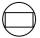The rectangle ABCD has side lengths a = 40 mm and b = 30 mm and is circumscribed by a circle k. Calculate approximately how many cm is circle long.
• PrismThree cubes are glued into a prism. The sum of the lengths of all its edges is 115 cm. What is the length of one edge of the original cube?
• A rectangle's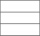A rectangle's length is 3 times more than twice its width. If the perimeter of the rectangle is 60 feet. What is the length of the rectangle
• An architectAn architect makes a model of a new house. The model shows a tile patio in the backyard. In the model each tile has length of 1/2 inch and width of 1/6 inch. The actual tiles have length of 2/3 feet and width 2/9 feet. What is the ratio of the length of a
• Rectangle 45The perimeter of a rectangle is 60cm. If the length of the rectangle is 20cm. a)find the width b)find the area.
• The rectangleThe rectangle has a circumference of 32 m. One side is 2 m longer than the other side. Calculate the side lengths of this rectangle.
• Area and perimeter 2Find the area and the perimeter of a rectangle of length 45 1/2 cm and breadth 16 2/3 cm.
• Rectangle A2dim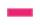Calculate the side of the rectangle, if you know that its area is of 2590 m2 and one side is 74 m.
• The perimeter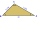The triangle has one side 5 cm long and the another 11 cm long. What can be the smallest and what is the largest perimeter?
• Sides of a rectangleThe dimensions of a rectangle are in a 4:12 ratio. If the shorter side length is 12 cm, what is the length of the longer side in centimetres?